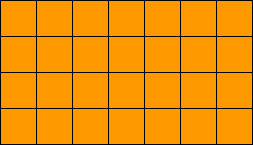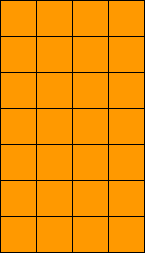Quandaries and Queries 3rd Grade math-- question 4x7 = 28 How many arrays can this have? How do you determine the arrays??? Jan Hi Jan, Since this is a grade 3 question I expect that what is wanted is the number of ways you can arrange 28 squares into a rectangular array. For example you have 47 = 28 which I see asArithematically the task is to write 28 as a product of integers in as many ways as possible. The number of arrays is 12 if you agree that 74 = 28 gives a different array than the one above.Penny Go to Math Central